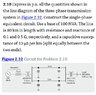# Three Phase Per Unit System - Mark up, represent as single phase equiv

• Evari5te

#### Evari5te

Homework Statement
Express in p.u. all the quantities shown in the line diagram of the three-phase transmission system in Figure 2.32.

Construct the single-phase equivalent circuit.

Use a base of 100 MVA.

The line is 80 km in length with resistance and reactance of 0.1 and 0.5 Ω, respectively, and a capacitive susceptance of 10 μS per km (split equally between the two ends).
Relevant Equations
##Value_{per~unit} = \frac{Actual~Value~(any~Unit)}{Base~or~Reference~Value~(in~the~same~unit)}##

For a three phase system:
##S_{base} = V_{base}.I_{base}.\sqrt(3)##

##Z_{base} = \frac{\frac{V_{base}}{\sqrt{3}}}{I_{base}}=\frac{\frac{V_{base}}{\sqrt{3}}}{\frac{S_{base}}{V_{base}.\sqrt{3}}}=\frac{V^2_{base}}{S_{base}}##

##Z_{p.u~(new~base)} = Z_{p.u~(old~base)}\times\frac{S_{new~base}}{S_{old~base}}##

Where S is a rated power selected to be a base or reference quantity, and a Voltage is usually selected as a second base quantity and from these two all other base quantities such as impedance, ##\mathbf{Z_b}## and current ##\mathbf{I_b}## are derived.
I'm seeking some reassurance I've understood the question since there are no answers given in the book and I'm getting a bit tied up in knots.

What I am struggling with most is there are three per unit values for impedance already noted on the diagram without qualifying what ##Z{base}## is or what base quantities were selected from which ##Z_{base}## can be computed.

If I assume that the original per unit impedance (or resistance) given of the generator is derived from its rated power and export voltage then##S_b = 500MVA## and ##V_b = 22 kV##

then the stated ##Z_{p.u.}## of the generator of 2 would be against a ##Z_{base} = \frac{V^2_{base}}{S_{base}} = \frac{2.2^2\times10^8}{5\times10^8} = 0.968\Omega##

The actual Z of the generator would then be ##2\times0.968 = 1.936\Omega##.

While the question gives direction to use a base of 100 MVA it does not specify a base voltage to use so I thought it would make sense to select 400 kV as being common to both transformers in which case both per unit reactances could be used if scaled by 100/500 to reflect the change of base MVA from 500 MVA to 100 MVA.

If I am correct about the original assumption of the generator per unit impedance being based on 500 MVA and 22 kV, giving actual impedance of ##1.936 \Omega## then a new base impedance with reference quantities 400 kV and 100 MVA will be:

##Z_{base~(new)} = \frac{4^2\times10^{10}}{10^8} = 1.6\times10^3\Omega##

and

##Z_{p.u} = \frac{1.936}{1.6\times10^3}=1.21\times10^{-3}\Omega_{p.u}##

But this isn't looking good - selecting 400 kV which is x18 bigger than 22 kV, before squaring it and a new MVA x5 less is leading to a base impedance orders of magnitude bigger and hence a new per unit impedance that is very small....

While the per unit reactance of each of the transformers will be the same whichever of the primary or secondary voltages might be selected as a base, they have dissimilar voltage combinations.

I am not sure if it follows that perhaps it doesn't matter which of the three voltages 22 kV, 400 kV or 11 kV was used as a base simply because they share one common voltage of 400 kV and are each related by turns ratio.

But surely it does matter to know which voltage is or was used as reference when it comes to the line R, X and B, since I will need to compute ##Z_{base}## for ##S_b = 100 MVA##and will get three different answers depending on which voltage level is selected as a reference, Vb. I will also need to be consistent with whatever reference values was used to compute the generator per unit resistance.

In the end I will assume that 22 kV was used as a base quantity along with 500 MVA to compute Zbase for the given generator per unit resistance and will continue to use it so I only need to scale all impedances by the change in ##S_b## from 500 MVA to 100 MVA.

This gives

Generator rating:##500\times0.2 = 5 MVA_{p.u}##
Generator resistance: ##2\times 0.2 = 0.4\Omega_{p.u}##
Step up transformer reactance: ##0.1\times0.2 = 0.02\Omega_{p.u}##
Step down transformer reactance: ##0.15\times 0.2 = 0.03\Omega_{p.u}##
Line Resistance R: ##\frac{0.1}{0.968}\times0.2 = 0.0207 \Omega_{p.u}##
Line Reactance X:=##\frac{0.5}{0.968}\times0.2 = 0.1033\Omega_{p.u}##
Line Susceptance B: = ##80\times10\times0.968\times0.2=154\mu~S = 0.154~ S##

Does this sound reasonable?

#### Attachments

•chapter 2_problem2_10.JPG
52 KB · Views: 15
It does sound reasonable. However, it is important to check your calculations and make sure that the values you have computed are correct. Additionally, make sure that the base quantities you have chosen (500 MVA and 22 kV) are consistent with the original per unit resistance of the generator.